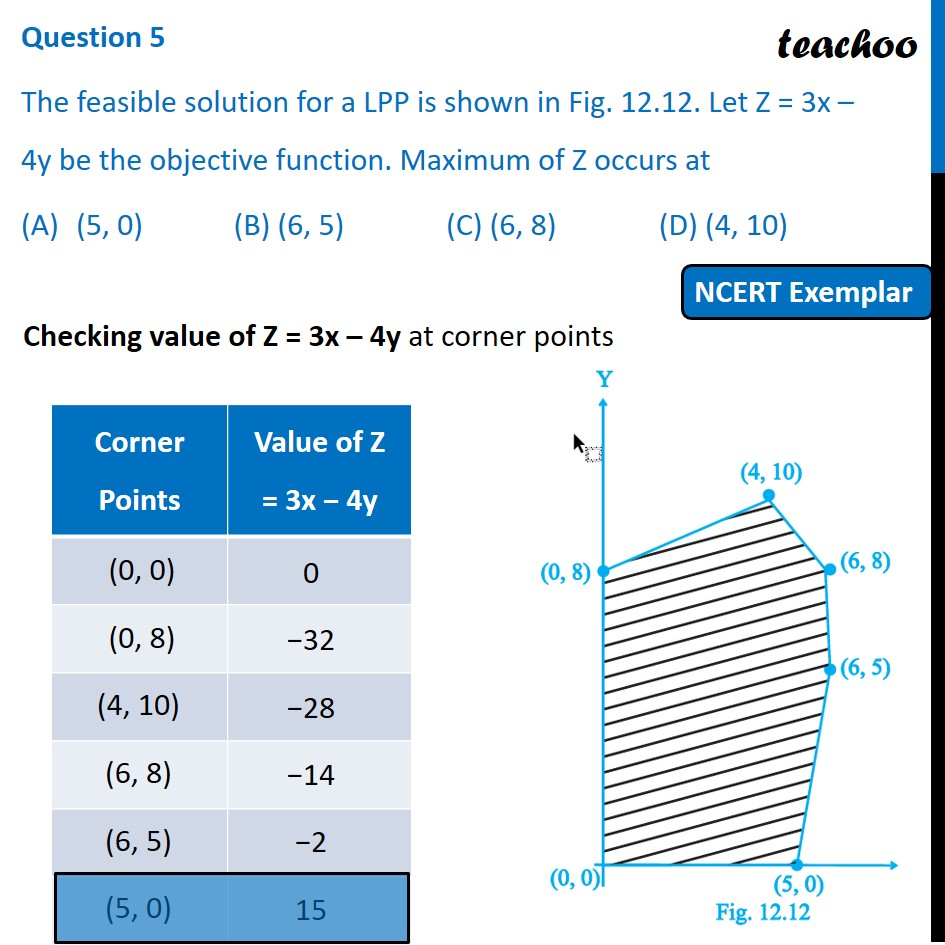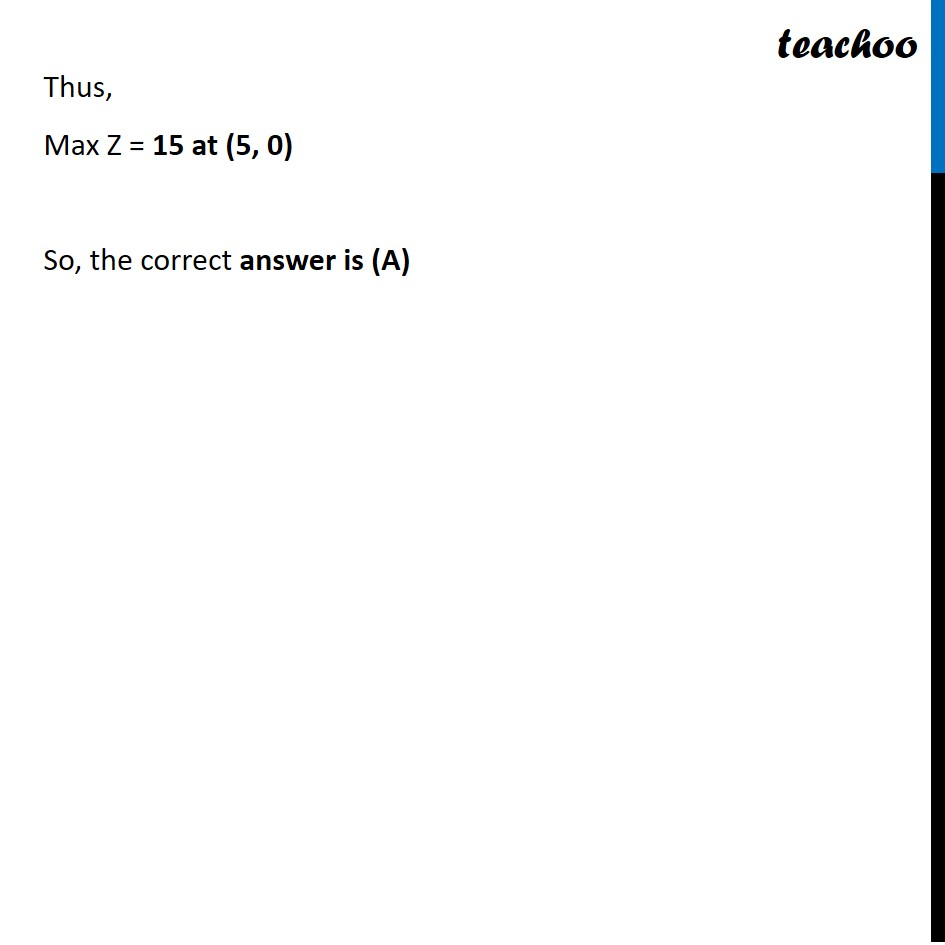NCERT Exemplar - MCQs

Chapter 12 Class 12 Linear Programming
Serial order wise

## (C) (6, 8)             (D) (4, 10)

This question is similar to Question 38 Choice 2 - Sample Paper 2021 Class 12 - Linear ProgrammingLearn in your speed, with individual attention - Teachoo Maths 1-on-1 Class

### Transcript

Question 5 The feasible solution for a LPP is shown in Fig. 12.12. Let Z = 3x – 4y be the objective function. Maximum of Z occurs at (5, 0) (B) (6, 5) (C) (6, 8) (D) (4, 10) Checking value of Z = 3x – 4y at corner points Thus, Max Z = 15 at (5, 0) So, the correct answer is (A)